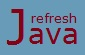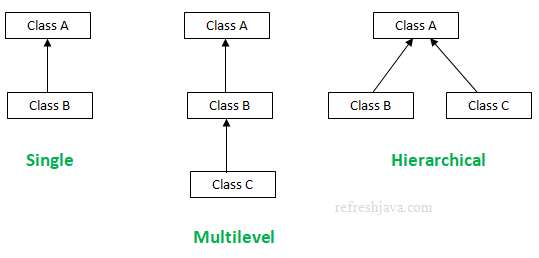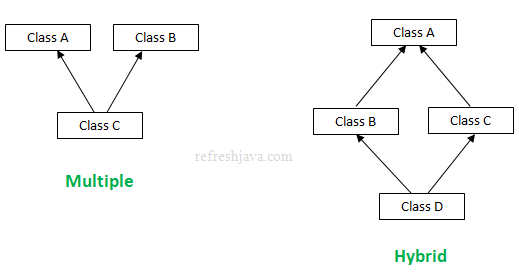# Different Types of Inheritance in Java

There are different types of inheritance supported by java. The programmers can use any of them as per the requirement. Before you start this tutorial, you should refer Inheritance in Java tutorial first if you are not aware about what inheritance in java is. The different types of inheritance supported in java are :

• Single inheritance
• Multilevel inheritance
• Hierarchical inheritance
• Multiple inheritance(Supported using interfaces, not by classes)
• Hybrid inheritance(Mix of two or more of above inheritance types)## Single level Inheritance in Java

When a class inherits only one another class, we call it single inheritance or single level inheritance. This is the simplest form of inheritance as it involves only two classes. Below program shows an example of single inheritance.

### Single inheritance program in Java

``` `class` A {
`void` add(`int` a, `int` b) {
System.out.println(a +`"+"` +b+`" = "`+(a+b));
}
}

`class` B `extends` A {
`void` subtract(`int` a, `int` b) {
System.out.println(a +`"-"` +b+`" = " `+(a-b));
}
`public static void` main(String args []) {
B obj = `new` B();
obj.subtract(20,10);
}
}```

Output:

```5+10 = 15
20-10 = 10```

## Multilevel Inheritance in Java

When three or more than three classes inherits in same chain or level, we call it multilevel inheritance. The number of classes in multilevel inheritance is not limited to three classes, it can go up to any number of classes in the same level or inheritance chain.

In multilevel inheritance the class at any position(level) can access the features of all the classes which are on top of that level. For example the class at bottom of chain will be able to access the features of class which is on top of that chain. The program below shows an example of multilevel inheritance.

### Multilevel inheritance in java Program

``` `class` A {
`void` add(`int` a, `int` b) {
System.out.println(a +`"+"` +b+`" = "`+(a+b));
}
}

`class` B `extends` A {
`void` subtract(`int` a, `int` b) {
System.out.println(a +`"-"` +b+`" = " `+(a-b));
}
}

`class` C `extends` B {
`void` multiply(`int` a, `int` b) {
System.out.println(a +`"*"` +b+`" = " `+(a*b));
}
`public static void` main(String args []) {
C obj = `new` C();
obj.subtract(20,10);
obj.multiply(10,20);
}
}```

Output:

```5+10 = 15
20-10 = 10
10*20 = 200```

## Hierarchical Inheritance in Java

When two or more than two classes inherits a single class, we call it hierarchical inheritance. In java a class can be inherited by any number of classes, so in hierarchical inheritance, there can be any number of child classes, it's not limited to two classes only. The program below shows an example of hierarchical inheritance.

### Hierarchical inheritance program in Java

``` `class` A {
`void` add(`int` a, `int` b) {
System.out.println(a +`"+"` +b+`" = "`+(a+b));
}
}

`class` B `extends` A {
`void` multiply(`int` a, `int` b) {
System.out.println(a +`"*"` +b+`" = " `+(a*b));
}
}

`class` C `extends` A {
`void` divide(`int` a, `int` b) {
System.out.println(a +`"/"` +b+`" = " `+(a/b));
}
}

`class` Test {
`public static void` main(String args []) {
B obj = `new` B();
obj.multiply(20,10);

C obj2 = `new` C();
obj2.divide(20,10);
}
}```

Output:

```5+10 = 15
20*10 = 200
20+30 = 50
20/10 = 2```

## Multiple Inheritance in Java

When a class inherits the features from more than one parent, we call it multiple inheritance. Multiple inheritance in java is not supported using classes, which means a class can not extend more than one class.

Multiple inheritance in java is supported through interfaces only, which means a class can inherit the features(specially `default` and `static` methods from java 8 onwards) from more than one interfaces. Java allows a class to implement any number of interfaces. The program below shows an example of multiple inheritance.

### Multiple inheritance program in Java

``` `interface` A {
`public void` print();
`default void` add(`int` a, `int` b) {
System.out.println(a +`"+"` +b+`" = "`+(a+b));
}
}

`interface` B {
`public void` print();
`default void` subtract(`int` a, `int` b) {
System.out.println(a +`"-"` +b+`" = " `+(a-b));
}
}

`class` C `implements` A, B {
`public void` print() {
System.out.println(`"Testing multiple inheritance in Java"`);
}
`public static void` main(String args []) {
C obj = `new` C();
obj.print();
obj.subtract(20,10);
}
}```

Output:

```Testing multiple inheritance in Java
20+10 = 30
20-10 = 10```

The above program will work if you are using java 8 or onward. If you are using any lower version, then you need to remove the default methods and it's calling from class `C` to make it work. There are couple of points to note about multiple inheritance through interfaces :

• If a class implements two interfaces which have same name variables and if the class needs to access such variables, then they must be accessed by interface name. The same applies with `static`(java 8 onwards) methods as well.
• If a class implements two interfaces which have same `default`(java 8 onwards) methods, then that method must be overridden in implementing class.
• A class cannot implement two interfaces that have method with same name but different return type.

To know more key points about interfaces refer interfaces key points tutorial.

## Why Java does not support multiple inheritance through classes ?

This is a design decision taken by the creators of java. To make java simple and reduce the complexity, multiple inheritance is not supported in java.

For example consider a scenario where we have three classes A, B, and C. The class C inherits class A and B. If classes A and B have same name method(or variable), now if you try to call the same method with object of child class C, the object will get confused, since there will be ambiguity to call the method of class A or class B. So ambiguity is one of the main reason why java does not support multiple inheritance.

## Hybrid Inheritance in Java

Hybrid inheritance, as the name itself suggests, it is a mix of two or more inheritance types given above. The hybrid inheritance diagram given in the beginning of this tutorial is a mix of hierarchical and multiple inheritance but it's not limited to that only, it may contain the mix of any other inheritance types as well.

If hybrid inheritance contains multiple inheritance, then it won't be supported through classes, as java doesn't support multiple inheritance using classes. It would be supported through interfaces only.

### Can we access all variables and features of parent class in child class ?

No, the accessibility of parent class features inside child class is also decided by access modifier. The child class can access only those variables/features of parent class which has an access modifier that allows it to be accessed outside the class. For example a `private` variable or method in parent class won't be accessible inside child class.

★★★
• Since multiple inheritance is not supported, so if a class inherits another class then it wont extend the Object class of java. In this case parent class will inherit the Object class.
• The classes involved in any inheritance type can be in same or different packages.
• C++ , Common lisp and few other languages supports multiple inheritance.
• Multiple Inheritance is not used very often in software projects.